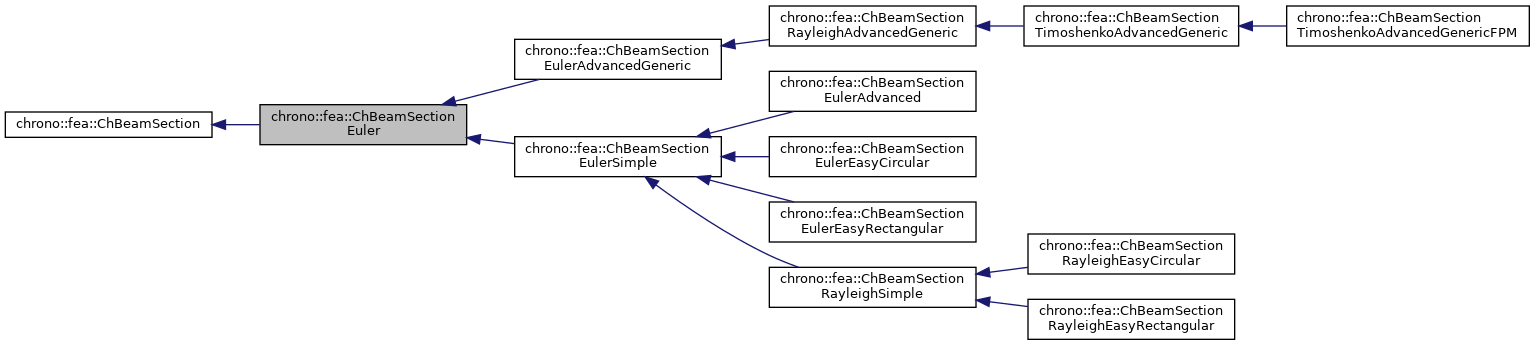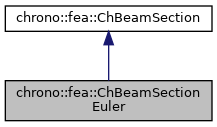chrono::fea::ChBeamSectionEuler Class Referenceabstract

## Description

Base class for all constitutive models of sections of Euler beams.

To be used with ChElementBeamEuler. For practical purposes, either you use the concrete inherited classes like ChBeamSectionEulerSimple, ChBeamSectionEulerAdvanced etc., or you inherit your class from this.

#include <ChBeamSectionEuler.h>

Inheritance diagram for chrono::fea::ChBeamSectionEuler:[legend]
Collaboration diagram for chrono::fea::ChBeamSectionEuler:[legend]

## Public Member Functions

virtual double GetAxialRigidity () const =0
Gets the axial rigidity, usually A*E, but might be ad hoc.

virtual double GetXtorsionRigidity () const =0
Gets the torsion rigidity, for torsion about X axis at elastic center, usually J*G, but might be ad hoc.

virtual double GetYbendingRigidity () const =0
Gets the bending rigidity, for bending about Y axis at elastic center, usually Iyy*E, but might be ad hoc.

virtual double GetZbendingRigidity () const =0
Gets the bending rigidity, for bending about Z axis at elastic center, usually Izz*E, but might be ad hoc.

virtual double GetSectionRotation () const =0
Set the rotation of the Y Z section axes for which the YbendingRigidity and ZbendingRigidity are defined.

virtual double GetCentroidY () const =0
Gets the Y position of the elastic center respect to centerline.

virtual double GetCentroidZ () const =0
Gets the Z position of the elastic center respect to centerline.

virtual double GetShearCenterY () const =0
Gets the Y position of the shear center respect to centerline.

virtual double GetShearCenterZ () const =0
Gets the Z position of the shear center respect to centerline.

virtual double GetMassPerUnitLength () const =0
Get mass per unit length, ex.SI units [kg/m].

virtual double GetInertiaJxxPerUnitLength () const =0
Get the Jxx component of the inertia per unit length (polar inertia) in the Y Z unrotated reference frame of the section at centerline. More...

virtual void ComputeInertiaMatrix (ChMatrixNM< double, 6, 6 > &M)=0
Compute the 6x6 sectional inertia matrix, as in {x_momentum,w_momentum}=[Mm]{xvel,wvel} The matrix is computed in the material reference (i.e. More...

virtual void ComputeQuadraticTerms (ChVector<> &mF, ChVector<> &mT, const ChVector<> &mW)=0
Compute the values of inertial force & torque depending on quadratic velocity terms, that is the gyroscopic torque (null for Euler beam as point-like mass section, might be nonzero if adding Rayleigh beam theory) and the centrifugal term (if any). More...

void SetArtificialJyyJzzFactor (double mf)
The Euler beam model has no rotational inertia per each section, assuming mass is concentrated on the centerline. More...

double GetArtificialJyyJzzFactor ()

void SetBeamRaleyghDamping (double mr)
Set the Rayleigh damping ratio r (as in: R = r * K ), to do: also mass-proportional term.

double GetBeamRaleyghDamping ()Public Member Functions inherited from chrono::fea::ChBeamSection
void SetDrawShape (std::shared_ptr< ChBeamSectionShape > mshape)
Set the graphical representation for this section. More...

std::shared_ptr< ChBeamSectionShapeGetDrawShape () const
Get the drawing shape of this section (i.e.a 2D profile used for drawing 3D tesselation and visualization) By default a thin square section, use SetDrawShape() to change it.

void SetDrawThickness (double thickness_y, double thickness_z)
Shortcut: adds a ChBeamSectionShapeRectangular for visualization as a centered rectangular beam, and sets its width/height. More...

void SetDrawCircularRadius (double draw_rad)
Shortcut: adds a ChBeamSectionShapeCircular for visualization as a centered circular beam, and sets its radius. More...

void SetCircular (bool ic)
OBSOLETE only for backward compability More...

## Protected Attributes

double JzzJyy_factor

## ◆ ComputeInertiaMatrix()

 virtual void chrono::fea::ChBeamSectionEuler::ComputeInertiaMatrix ( ChMatrixNM< double, 6, 6 > & M )
pure virtual

Compute the 6x6 sectional inertia matrix, as in {x_momentum,w_momentum}=[Mm]{xvel,wvel} The matrix is computed in the material reference (i.e.

it is the sectional mass matrix)

Parameters
 M 6x6 sectional mass matrix values here

## ◆ ComputeQuadraticTerms()

 virtual void chrono::fea::ChBeamSectionEuler::ComputeQuadraticTerms ( ChVector<> & mF, ChVector<> & mT, const ChVector<> & mW )
pure virtual

Compute the values of inertial force & torque depending on quadratic velocity terms, that is the gyroscopic torque (null for Euler beam as point-like mass section, might be nonzero if adding Rayleigh beam theory) and the centrifugal term (if any).

All terms expressed in the material reference, ie. the reference in the centerline of the section.

Parameters
 mF centrifugal term (if any) returned here mT gyroscopic term returned here mW current angular velocity of section, in material frame

## ◆ GetInertiaJxxPerUnitLength()

 virtual double chrono::fea::ChBeamSectionEuler::GetInertiaJxxPerUnitLength ( ) const
pure virtual

Get the Jxx component of the inertia per unit length (polar inertia) in the Y Z unrotated reference frame of the section at centerline.

Note: it automatically follows Jxx=Jyy+Jzz for the polar theorem. Also, Jxx=density*Ixx if constant density.

## ◆ SetArtificialJyyJzzFactor()

 void chrono::fea::ChBeamSectionEuler::SetArtificialJyyJzzFactor ( double mf )
inline

The Euler beam model has no rotational inertia per each section, assuming mass is concentrated on the centerline.

However this creates a singular mass matrix, that might end in problems when doing modal analysis etc. A solution is to force Jyy and Jzz inertials per unit lengths to be a percent of the mass per unit length. By default it is 1/500. Use this function to set such factor. You can also turn it to zero. Note that the effect becomes negligible anyway for finer meshing.

The documentation for this class was generated from the following file:
• /builds/uwsbel/chrono/src/chrono/fea/ChBeamSectionEuler.h